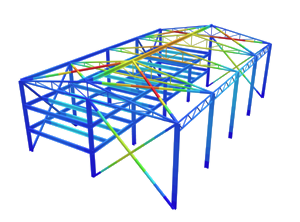SkyCiv Documentation

Your guide to SkyCiv software - tutorials, how-to guides and technical articles

1. Home
2. Tutorials
3. Beam Tutorials
4. How to Calculate Shear Force Diagrams?

# How to Calculate Shear Force Diagrams?

## How to find a Shear Force Diagram (SFD) of a Simple Beam

In this tutorial, we will look at calculating the shear force diagram of a simple beam. This is an important concept to understand, as shear force is something a beam will need to be checked for, for a safe design.

### What is a Shear Force?

Firstly, what is a shear force? A shearing force occurs when a perpendicular force is applied to static material (in this case a beam). Think of a knife cutting through a carrot. Imagine the beam is the carrot and a point load is the knife. As the knife applies a downward force, it cuts (or shears) the carrot. These forces occur along numerous points of a beam, and it is important to determine where these shears are at the greatest points as this may be where a beam fails.

To calculate the shear forces of a beam, follow the following simple steps (as hand calculated by SkyCiv Beam Software). But first, a few things to note with this process:

#### Start with the far left side of the beam

If there is an upward force (i.e a support) then the SFD will start at this force above the x-axis. If there is a downward point load and no support than the shear force diagram will start as a negative at the value of the point load.

#### Move across the beam

As you come across loads, you simply add (or subtract) these loads from the value you already have, keeping a cumulative total.

It is much easier to understand when considering an example of how to calculate a Shear Force Diagram. So let’s consider the following example to calculate the shear force diagram of a beam: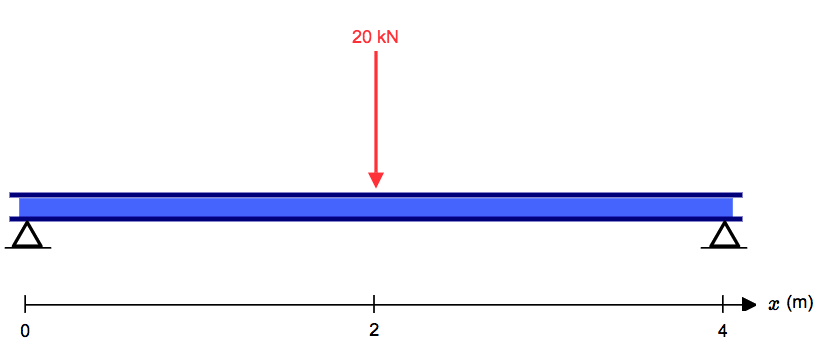Source: SkyCiv Beam

## Step 1:

After you calculate the reactions at supports at A and B, start the Shear Force Diagram at the first value of the force acting on the beam. In this case, it is a +10kN due to the reaction at point A: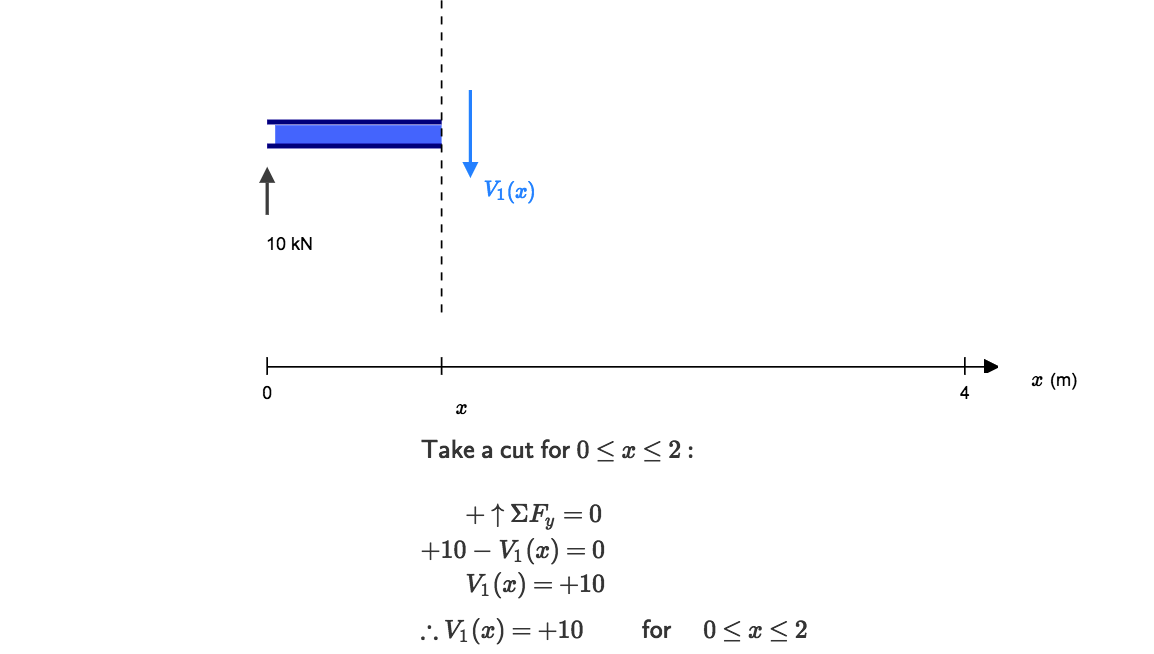## Step 2:

Keep moving across the beam, stopping at every load that acts on the beam. When you get to a load, add to the Shear Force Diagram by the amount of the force. In this case we have come to a negative 20kN force, so we will minus 20kN from the existing 10kN. i.e. 10kN – 20kN = -10kN.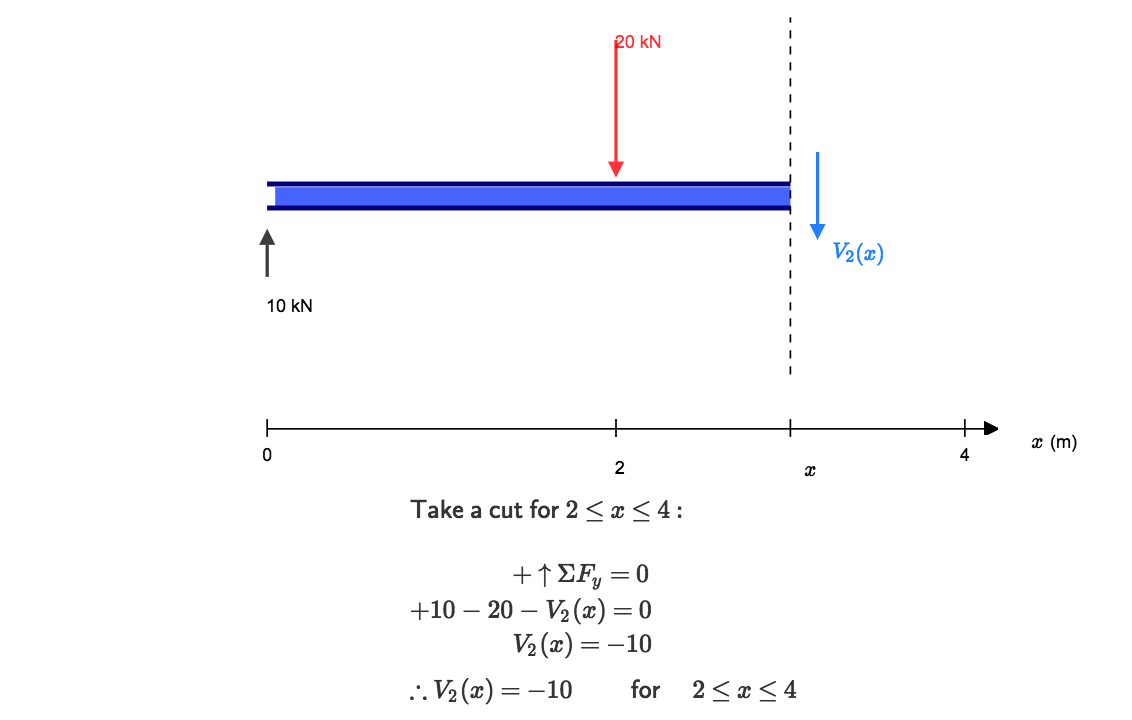## Step 2 (Repeated):

Moving across the beam again, we come to another force; a positive 10kN reaction at support B. Again, add this +10kN to the shear force diagram (which is currently at -10kN) which will bring us to a shear force of 0. Since we are at the end of the beam, we will go no further and we have our final Shear Force Diagram (SFD):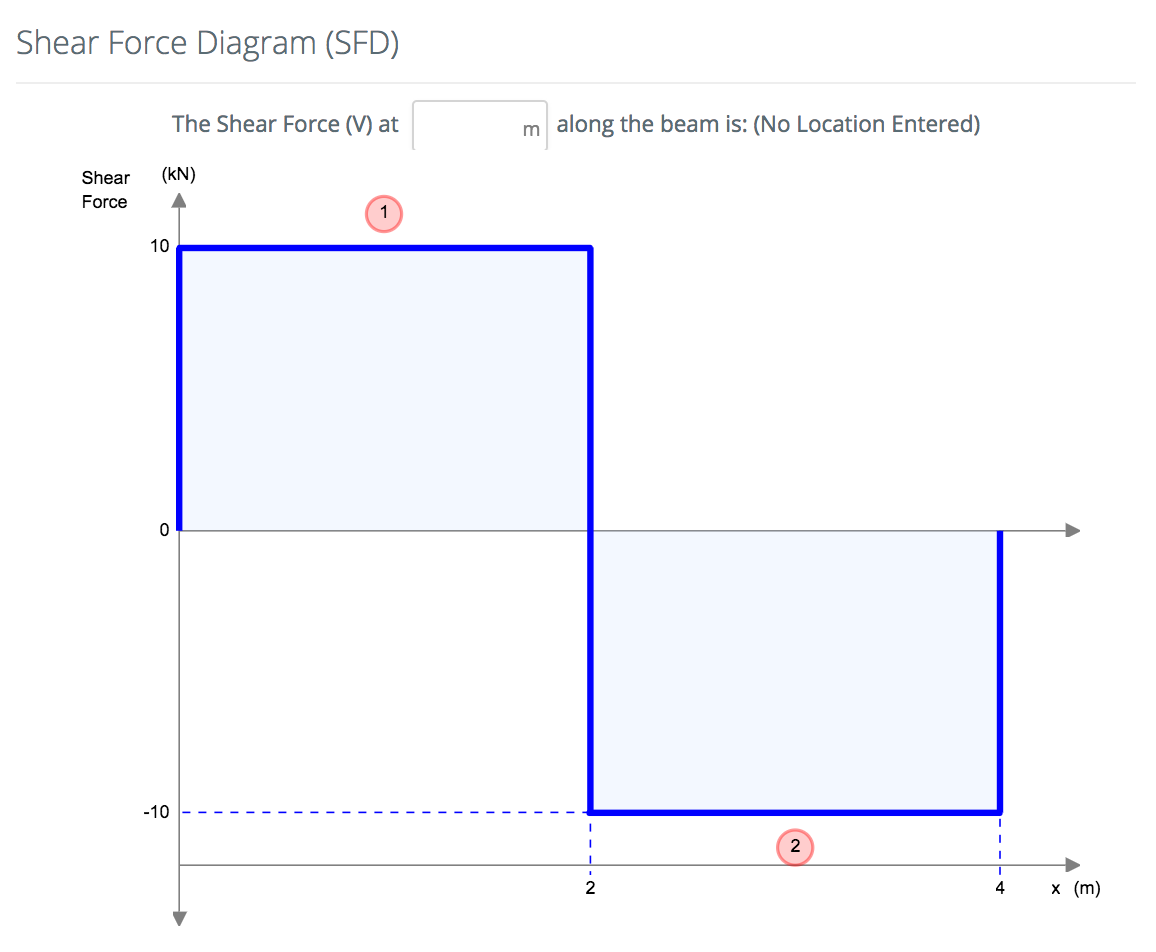Source: SkyCiv Beam

Things to keep in mind:

• The area under the SFD above the x axis should equal the area between the x-axis and the SFD below the x axis. i.e the area should sum to zero. Check this is true in our above example.
• Any points where the SFD cross the x-axis, will be a max or min Bending Moment
• The SFD should always equal zero at both ends

Visit the next step: How to calculate Bending Moment Diagrams of Simply Supported Beams.